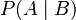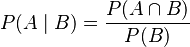# Conditional probability facts for kids

Kids Encyclopedia Facts

In probability theory, conditional probability is the probability of an event occuring, given that another event has occurred. Usually this is written as$P(A\mid B)$. This is read as "probability of A given B". The two events need not be related. They also need not occur at the same time. In the most general case,$P(A\mid B) = \frac{P(A\cap B)}{P(B)}$

Expressed in words: The conditional probability of A occurring given B is the probability of both events occurring, divided by the probability of B occurring. As a division by zero is not defined, the probability of B occurring must not be zero.

## Images for kidsConditional probability Facts for Kids. Kiddle Encyclopedia.